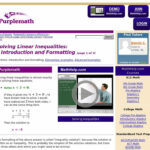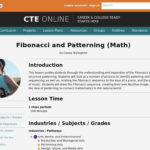# Tag Archives: algebra#### Solving Linear Inequalities: Introduction and Formatting

September 14th, 2017

From Elizabeth Stapel at Purplemath, this module helps students understand how to solve linear inequalities with an introduction to the topic using both inequality notation and a graphic representation to express the inequality.#### Fibonacci and Patterning (Math)

September 14th, 2017

This lesson guides students through the understanding and expansion of the Fibonacci series and recursive patterning. Students will look at a number of pictures to identify pattering and sequencing, as well as, relating the Fibonacci sequence to the keys of a piano, and the production of music. Students will draw the Fibonacci sequence, creating their […]